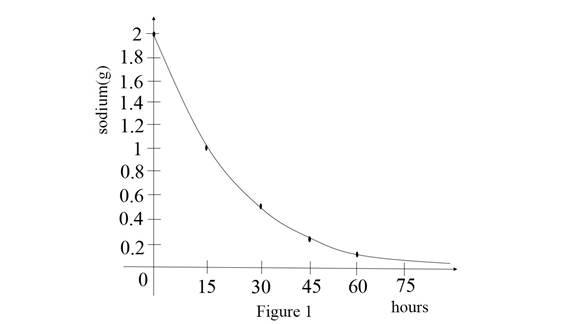# The amount remaining isotope of sodium after 60 hours.### Single Variable Calculus: Concepts...

4th Edition
James Stewart
Publisher: Cengage Learning
ISBN: 9781337687805### Single Variable Calculus: Concepts...

4th Edition
James Stewart
Publisher: Cengage Learning
ISBN: 9781337687805

#### Solutions

Chapter 1.5, Problem 32E

(a)

To determine

## To find: The amount remaining isotope of sodium after 60 hours.

Expert Solution

The amount remaining isotope of sodium after 60 hours is 0.125g.

### Explanation of Solution

Given:

The half-life of sodium is 15 hours.

The mass of sample sodium = 2g.

Calculation:

Let m0 be the initial mass of sodium.

That is m0=2g .

After 15 hours the amount of sodium is m1=12m0 .

Substitute 2g for m0 in m1 ,

m1=12(2)m1=1g

After 30 hours the amount of sodium m2=12m1

Substitute 1g for m1 in m2 ,

m2=12(1)m2=0.5g

After 45 hours the amount of sodium is m3=12m2

Substitute 0.5g for m2 in m3 ,

m3=12(0.5)m3=0.25g

After 60 hours the amount of sodium is m4=12m3

Substitute 0.25g for m3 in m4 ,

m4=12(0.25)m4=0.125g

Therefore, the amount sodium after 60 hours is 0.125g.

(b)

To determine

### To find: The amount of sodium after t hours.

Expert Solution

After t hours amount of sodium is mt=2t15(2) .

### Explanation of Solution

Calculation:

Consider half –life sodium amount in 15 hours = 12m0 .

Where m0=2g .

After t=15xhours .where x be variable.

Therefore after t hours amount of sodium as follows,

mt=12x(m0) (1)

Substitute t15 for x and 2 for m0 in equation (1),

mt=12t15(2)mt=2t15(2)

Therefore, after t hours amount of sodium is mt=2t15(2) .

(c)

To determine

### To estimate: The remaining amount of sodium after 4 days.

Expert Solution

After 4days the amount of sodium is m96=0.0236g .

### Explanation of Solution

Given:

Consider after 15 hours the amount of sodium = 1g.

m0=2g .

Calculation:

After 15 hours the amount of sodium is m1=12m0 .

By using part (b) solution mt=2t15(2) for t hours.

Obtain the amount after 4 days that means 96 hours.

Substitute 96 for t in mt=2t15(2) ,

m96=29615(2)m96=26.4(2)m96=0.0118(2)m96=0.0236g

Therefore, after 4 days the amount of sodium is m96=0.0236g .

(d)

To determine

### To estimate: The amount of bismuth reduced to 0.01g.

Expert Solution

The amount of sodium amount reduced to 0.01g for 38.2215 days.

### Explanation of Solution

Given:

The reduced amount of sodium = 0.01g.

Graph:

Let x axis for hours and y axis for sodium (g).

Use the online graphing calculator and draw the graphs for sodium level and hours as shown below Figure 1.Observe the graph an initial stage sodium amount is 2g.

Then after 15 hours the mass is reducing to 1g.

After 30 hours the mass is reducing to 0.5g.

After 45 hours mass is reducing to 0.25g.

After 60 hours mass is reducing to 0.125g.

Therefore, the mass of sodium is reducing when the hours are increasing.

Calculation:

From the graph, the general form of sodium after t hours mt=2t15(2) .

Substitute 0.01 for mt in mt=2t15(2) ,

0.01=2t15(2)0.012=2t150.005=2t15

Take naturel logarithm on both sides,

ln(0.005)=ln(2t15)ln(0.005)=t15ln(2)ln(0.005)ln(2)=t15t=15(ln(0.005)ln(2))

Simplify further,

t=15(5.29830.6931)t=15(7.6443)t=114.6645

Therefore, the amount of sodium reduced to 0.01g for 114.6645 hours.

### Have a homework question?

Subscribe to bartleby learn! Ask subject matter experts 30 homework questions each month. Plus, you’ll have access to millions of step-by-step textbook answers!